## Background Theory and Concepts of Illumination Design

Category: Product NewsThis blog provides the fundamentals of illumination systems, in particular, the background and a little theory of illumination systems. There are no lengthy derivations of theoretical equations, but rather a discussion on the fundamentals, such as "What makes a good illumination design?".

This blog provides useful concepts behind illumination design that help you set up the right system for your design needs.

#### Introduction

In this post, we’ll introduce the essential concepts before we start an illumination design. We explore the concepts that are useful for illumination design, such as the units of measure, the energy of the system, and the conservation of energy (étendue).

#### The intent of this blog (what it is and isn't)

This blog is not meant as a derivation of the various theories of optics that can be used in illumination design, but rather to present the underlying theory and concepts that a designer should be aware of when performing an illumination design. Complete derivations of the equations are not provided.

#### Some background theory and concepts of illumination design

Nonimaging optics, or non-sequential raytracing is often used for illumination. A different thought process is needed for most illumination designs.

Illumination was not developed based on mathematical formulae, like imaging optics. Traditional optics have long been based on imaging optics, where we trace few rays (much fewer than what represents the physical world). We use these rays to calculate first-order optics such as focal length, and third-order optics such as Seidel aberration theory. Since these optical properties require intense mathematical calculations, they do not require all of the rays to be traced, and only need a subset of the entire physical rays.

Nonimaging optics is a subset of optics that, compared to traditional imaging optics, do not attempt to form an image of an object. The primary objective of nonimaging optics is the transfer of light between the source and the illuminated target. The aim of a nonimaging optical system is the optimal transfer of the light source to the desired distribution on the target of illumination.

With the computing power that we have today, it is possible to trace millions (sometimes billions) of rays and saturate the illumination target to get a very close representation of the illuminated surface. Notice that the previous sentence bears no mention of any formulaic calculations, and is a near brute-force approach. It is more of a simulation than a derivation or calculation. Most times, the design is not based on an algorithm but intuition and heuristic results.

As stated above, the primary goal of an illumination design is the optimal transfer of the light source to the desired distribution on the target illumination. However, common optical engineering properties such as color, cost, and ease of manufacture also apply. It is harder to quantify the latter requirements as a merit function, for example. Even the desired distribution and total throughput or efficiency are difficult to quantify into a merit function compared to "the focal length = 50mm". A focal length (or MTF, spot size, or distortion), for example, is a fixed number. Whereas the uniformity of the target surface can be defined in several different ways.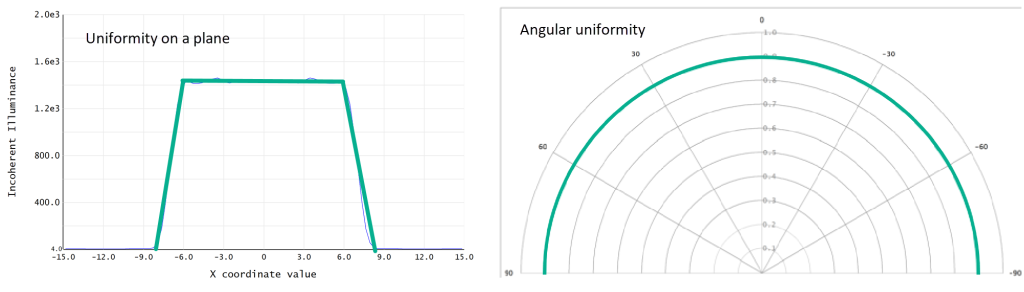Of course, a completely uniform surface is easily definable. However, is a better uniformly illuminated surface, A) a surface that is uniform in the center but has a drop-off in the corners, or B) a surface that has better-illuminated corners but with a doughnut or ripple-like distribution in an intermediary region? Unfortunately, the short answer is, it all depends on our Performance targets of illumination design. Due to the diversity of illuminations systems, it means that a set method and process of design can rarely cover all aspects of illumination systems. However, some fundamentals concepts are useful, or even essential, when performing an illumination design.

### Fundamental concepts that are useful in illumination design

#### Units of measure

There are two aspects to the unit of measure of an illumination system: radiometric quantities and photometric quantities. While radiometry is the measurement of electromagnetic radiation, including the visible light spectrum, photometry measures the response of the human eye to light. The distinction between these two units is essential when we are considering our illumination system. The terms may be confusing at first, but to summarize, the radiometric quantities are radiant flux Φ, irradiance E, radiant intensity I, and radiance L, while the photometric quantities are luminous flux Φ, illuminance E, luminous intensity I, and luminance L.

Below is a shorthand table for quick access to the illumination units.

 Radiometric Photopic Term Symbol Name Units Name Units Flux Φ Power Watts (W) Luminous flux lumens (lm) Flux/area E Irradiance W/m² Illuminance lm/m² or lux Flux/solid angle I Radiant intensity W/sr Luminous intensity lm/sr or candela (cd) Flux/area⋅solid angle L Radiance W/m²⋅sr Luminance lm/m²⋅sr or cd/m² or nit

Please note that the designations of ΦEIL are not universal, and sometimes PHJ, and N are used for the corresponding radiometric quantities, while FEI, and B are used for the corresponding photometric quantities. A complete explanation of the units of measure are provided in the Knowledgebase article Performance targets of illumination design.

#### Point sources

Some light sources are small compared to the optical system, and can be reduced to a point source for more straightforward calculations. For example, some small LEDs, most single-mode laser diodes (LDs), and some multi-mode LDs have a small surface area, and the source can be regressed into a point. If the light source can be reduced to a point, many calculations are more straightforward and involve less computer power for optimization and ray trace simulations. It is good practice to check the system to the actual size of the light source after a few or several iterations of the design, when the lens optimization has progressed enough that the size of the light source has a more significant impact.

Some simple optical systems are collimators, which is an optical device that generates parallel beams of light. We use a collimator to send light far away, in a distant direction. Some examples of collimator systems in application systems are flashlights, automotive headlamps, laser pointers, and the lighthouse lenses. A plano-convex lens can be a refractive collimating lens, while a paraboloid reflector can be a collimating reflector as well. Both can collimate a point source is the point source is placed at the focal point of the lens.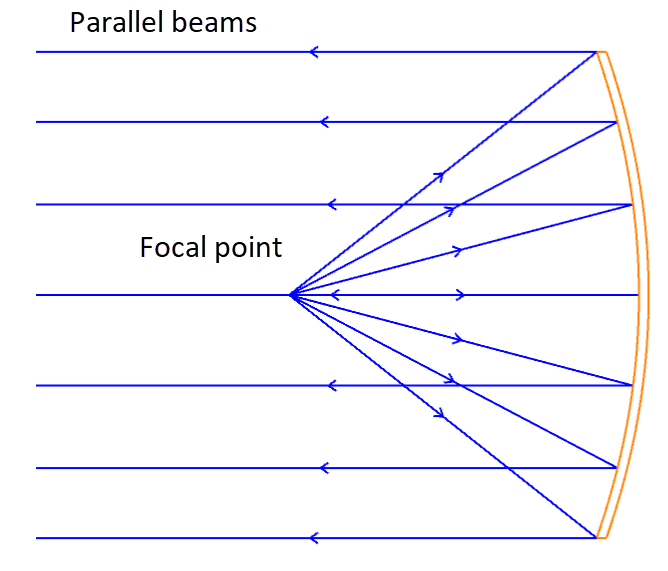When designing with a point source, we are not taking into account the size of the light source, so we must use care when choosing this approximation for our illumination solution.

### Defining the energy

#### The encircled energy of a light source

In a nonimaging or illumination optical system, when we eventually calculate the efficiency of the system, the encircled energy of the light source is the reference energy. Let's take an LED as our example for encircled energy, much like the example in the image below.Let's use a simple Lambertian distribution for our LED light source. A Lambertian distribution is a uniform luminance L regardless of the observation angle. Also, a Lambertian distribution changes in luminous intensity I as cos(θ), better known as Lambert's cosine law. The reference to the angle θ is the surface normal to the light source.The encircled energy is the amount of luminous flux Φ within the cone of light of angle θ. The luminous flux Φ can be calculated by integrating the product of the solid angle and the luminous intensity I. This integration allows us to find out how much light we need, to the extent of the angle that the light source illuminates.For a Lambertian source, if we only use ±45 degrees of the LED, we see that we only collect about 50% of the entire flux. To use 90% of the flux, we need to collect a light cone of ±71.6 degrees. Things get a little more complicated when we consider the size of the light source since it adds another parameter to consider.

#### Consideration of encircled energy: TIR lens

To utilize as much light as possible, we need to collect a large angle from the light source. With a solely refractive lens or a solely reflective lens, the size of the optical part becomes much too large for efficient collection of the light. For this case, both refractive and reflective properties are considered in the optical design.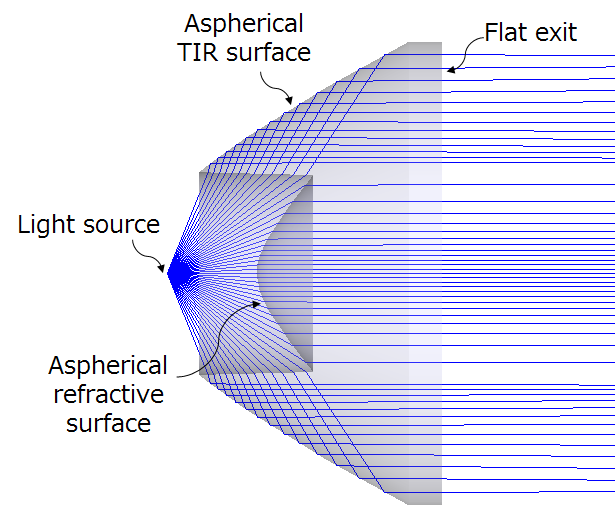(Reference: Pencil of Rays)

The front portion of the lens is refractive, while the side surfaces are reflective due to total internal reflection. Such a lens is a TIR lens. Although the TIR lens uses both refractive and reflective properties of the lens, it is typically called a TIR lens since a large part of the optical design relies on total internal reflection. As we can see from this example, maximizing the encircled energy of an illumination system uses design concepts that are nontrivial compared to imaging optics.

#### Uniform distribution

One concept of illumination design is the conservation of energy. Instead of randomly setting target values for optimization, we can apply this concept to an analytical design method for illumination systems. In the image below, a Gaussian distribution is transferred to a tophat distribution, albeit schematically. The distribution of energy is quantifiable for a Gaussian, since there is an analytical equation that expresses it. A top-hat distribution is also quantifiable, since it is a uniform distribution within a certain range. The lens in this example distributes the Gaussian distribution of the source into a flat, top-hat distribution.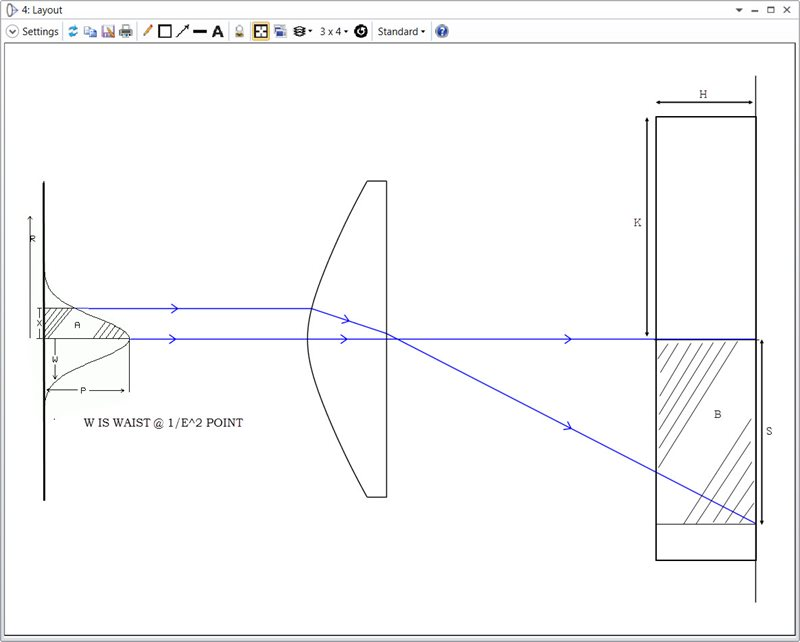For example, we can think of the luminous flux Φ of the optical system by two parameters:

If we ignore any other losses, the energy is conserved, then

ƒ1(θ) = ƒ2(r).

The above equation is a representation of θ and r, and can be used effectively in the early design phase when we are planning our illumination design concept. An example of the analytical design applied to a refractive beam shaper that converts a Gaussian intensity profile into a Top Hat profile can be found here.

### Étendue and the conservation of energy

#### How to interpret étendue in nonimaging and illumination design

Etendue or étendue is one of the most basic and most essential concepts in nonimaging and illumination optical system design. Although étendue is very useful in illumination design, it is often confused or misunderstood. The term étendue itself is directly translated into the word "extent" in English.

For our purposes, étendue means two things:

1. Explains the flux transfer characteristics of the optical system
2. Plays an integral role in the ability to shape the distribution of radiation at the target

Due to the fundamental property of étendue, for a source with a given radiance and a given optical system, the maximum flux that can be transmitted through the system is predetermined. The concept of étendue itself is a relatively new one, and in trying to define étendue and the conservation of étendue in optical illumination systems, a series of analogous terms are used that are often confused with étendue. Therefore, it is possible to interpret étendue in different ways (Étendue is sometimes described as merely the area multiplied by the solid angle, but other documentation provides the area multiplied by the radiant solid angle)

#### The mathematical interpretation of étendue

Mathematically, it is the product of the area and solid angle, and it is a fundamental property of the optical system that determines the amount of light that can get through the system. It is a way to determine the throughput of an optical system. Étendue is represented by the following equation.

Étendue ＝ π⋅A⋅NA²

where A is the cross-sectional area of the flux, π⋅NA² is the radiant solid angle, and NA = sinθ.

Étendue is conserved across the optical system, from the source to the detector. Much like water flowing down a river, we can represent the flow of water in a river by the cross-sectional area of the river multiplied by the speed of the water in the river. If the cross-sectional area of the area is doubled, the speed of the water is halved. Light has the same property: if the cross-sectional area of the light beam is doubled, then the solid angle is halved. Of course, this ignores any absorption or reflection in the optical system.

#### A practical example that illustrates étendue

Let's use a simple collimator example to illustrate the concept of étendue for two systems. For example, a square LED with a 0.3mm x 0.3mm illuminating area, with a radiant solid angle of ±90 degrees has an étendue of:

Étendue = π A NA²
= 3.14 × 0.3 × 0.3 × (sin90°)²
= 0.28mm² sr

If we make the area of the flux 100mm², the radiant solid angle is 0.0028 sr, and

NA＝√(0.0028/π) = 0.03,
∴ sinθ = 0.03,

and

θ＝asin(0.03)＝1.70°.

This means that if the illuminated area is 100mm², the parallelism of the rays is ±1.70° at best.

We can make a visual interpretation of the concept of étendue with a finite-sized light source and two collimators.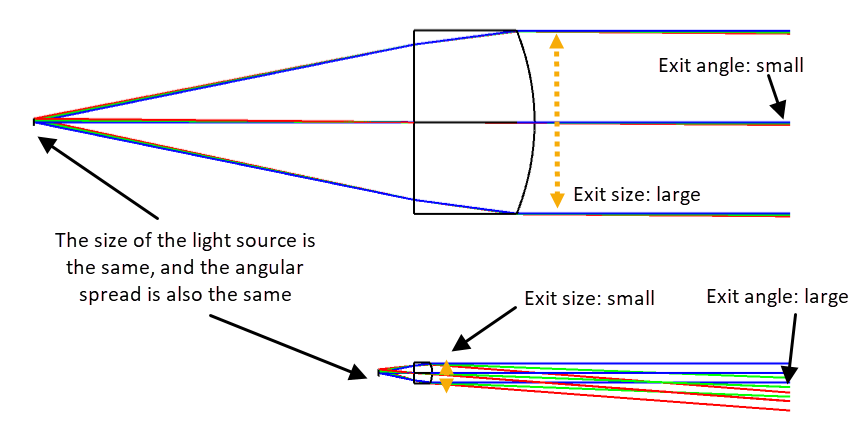• The smaller collimator has a focal length that is one-fourth of the larger collimator.

• The light source is the same for both examples

• the illuminating area of the source is the same

• the solid angle from the light source is the same

• The larger collimator has

• larger focal length

• smaller solid angle of exit rays

• larger cross-sectional area

• The smaller collimator has

• smaller focal length

• larger solid angle of exit rays

• smaller cross-sectional area

As we can see, there is a tradeoff between the size of the beam and the collimation. For this example, the possible landing points of the collimator illumination design are

1. A high-performance collimator that is large

2. A tiny collimator (and therefore compact/light/cheap lens) that does not collimate very well

3. Somewhere between the two

As lens designers, sometimes we get requests from clients to "design the smallest manufacturable lens with the smallest diverging collimated angle. With the concept of étendue and the conservation of étendue, we can make a reasonable assumption whether or not the request is an appropriate design goal or not, on the spot during the meeting. These kinds of quick estimates are invaluable during the initial phases of specification agreements between the lens designer and the client. You can set yourself apart from the average lens designer if you can think quickly on your feet during a face-to-face client meeting or a teleconference call, for example

Experience the power of Ansys Zemax optical design software for yourself. Request a free trial today!

Author:
Katsumoto Ikeda
Manager Application Engineering
Ansys